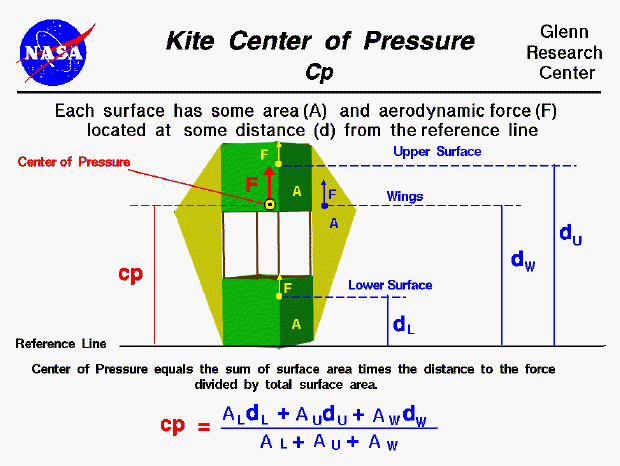The flight characteristics of a kite depend on the relative size of the forces which act on the kite and where those forces act on the kite. The forces include the aerodynamic lift, and drag which act through the center of pressure, the tension in the control line which acts through the bridle point, and the weight which acts through the center of gravity. We can predict the performance of a kite design if we can calculate all of these forces and locations.

The center of pressure is the average location of the aerodynamic forces acting on any object. To determine the stability and trim of the kite we need to be concerned with the magnitude of the aerodynamic forces and the location of the center of pressure. How would you determine the location of the center of pressure during design?

Calculating cp

You can calculate the center of pressure, but, in general, this is a complicated procedure requiring the use of calculus. This figure shows a simplified version of the center of pressure calculation. Because we are concerned with aerodynamic forces, we can neglect the effects of the frame of the kite, and concern ourselves only with the surfaces. Each surface has an area associated with it which you can calculate. Since each of the surfaces of a kite behaves like a thin, flat plate at low speed, we can determine the center of pressure of each surface as described below. Knowing the center of pressure of each surface we can compute the center of pressure for the kite as the area-weighted average of all the surfaces. For the winged box kite shown on the figure, we know the location, d, of the center of pressure and the area, A, of the wings, W, and the upper U and lower L surfaces. We choose as a reference line the bottom of the kite, so that the location of the kite center of pressure has the same reference as the center of gravity. (Note that normally the aerodynamic center is measured from the leading edge of a lifting airfoil; we are designating the aerodynamic center relative to the trailing edge).

A * cp = (A * d)U + (A * d)L + (A * d)W

A = (A)U + (A)L + (A)W

Component Cp Location

To simplify our analysis, we are going to assume that the aerodynamics of all of the surfaces of the kite behave like flat plates. We can then use techniques developed for airplane aerodynamics to determine our kite aerodynamics. In particular, for thin airfoils, it has been found that the aerodynamic force acts through a location called the aerodynamic center where the aerodynamic moment remains a constant with angle of attack. For a symmetric airfoil, like a flat plat, the magnitude of the aerodynamic moment is zero and the location of the aerodynamic center is 1/4 of the chord length back from the leading edge. In computing the center of pressure for the entire kite, we will assume that the aerodynamic force of each component acts through the mean aerodynamic center of each surface.

You can use the KiteModeler computer program to calculate values for the center of pressure of your design. Select "Design" mode and change the geometry of your kite using input sliders. The program calculates the center of pressure using the equation listed above and displays the output results.Beginner's Guide to Aerodynamics
Beginner's Guide to Propulsion
Beginner's Guide to Model Rockets
Beginner's Guide to Kites
Beginner's Guide to Aeronautics